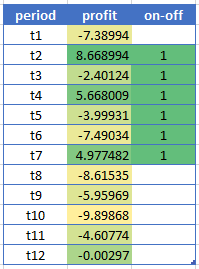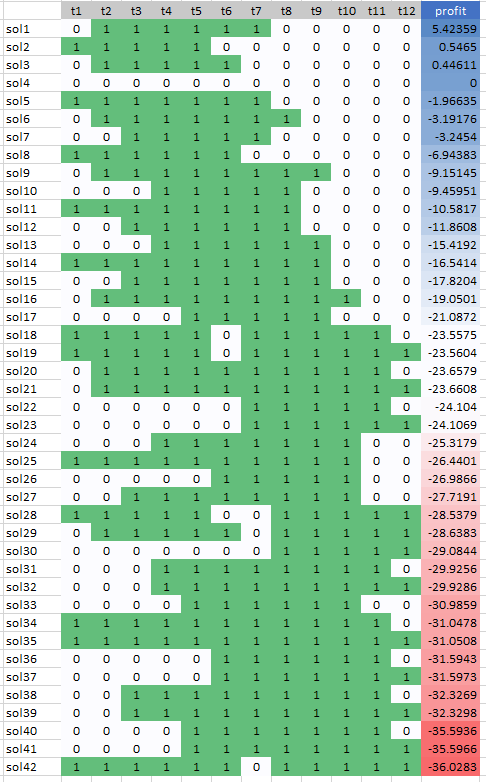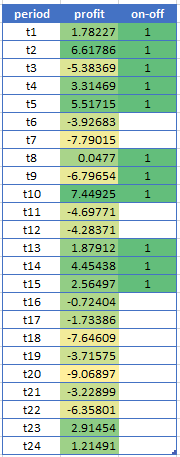## Wednesday, March 14, 2018

### production scheduling: Minimum up-time

In  a minimum up-time question is posted:

• Assume we have $$T=12$$ periods. If we produce in period $$t$$ we have a profit of $$c_t$$. Some $$c_t$$'s are negative.
• If we turn production on, it should remain on for at least $$n=5$$ periods.
• We want to maximize total profit.
• How can we write a constraint that captures this minimum up-time condition?

We can recognize production is turned on in period $$t$$ by observing $$x_{t-1}=0$$ and $$x_t=1$$ where $$x_t$$ is a binary variable indicating whether our production process is turned on or off. So our constraint can look like:
$x_{t-1}=0,x_{t}=1 \Longrightarrow \sum_{k=t}^{t+n-1} x_k = n\>\>\>\forall t$
In a MIP model we can translate this into:
$\sum_{k=t}^{t+n-1} x_k \ge n(x_t-x_{t-1}) \>\>\>\forall t$
This formulation is not completely obvious. We note that $$x_t-x_{t-1}$$ is zero or negative for all cases except $$x_{t-1}=0,x_{t}=1$$.

Some notes:
• Behavior near the boundaries is always important. E.g. we could assume $$x_0=0$$.
• Let's also assume $$x_{T+1}=0$$ so we need to finish our production run in the time horizon.
• The MIP constraint can also be written as: $\sum_{k=t+1}^{t+n-1} x_k \ge (n-1)(x_t-x_{t-1}) \>\>\>\forall t$ as we know $$x_t=1$$ when this thing kicks in.
• So this gives us 12 constraints for our $$T=12, n=5$$ problem.
• We can disaggregate the summation into individual constraints $x_k \ge x_t-x_{t-1}\text{ for } k=t+1,\dots,t+n-1$ This is tighter and may solve faster. Advanced solvers may use an "implied bound" cut to do this automatically (and smarter) .
A complete model can look like:
\begin{align} \max \>&\sum_t c_t x_t\\ &\sum_{k=t}^{t+n-1} x_k \ge n(x_t-x_{t-1}) \\ & x_t \in \{0,1\}\end{align}
I implemented this in GAMS. GAMS assumes that variables indexed outside their domain are zero. This means I don't have to do anything special for $$t=1$$ and for $$t\gt T-n$$.

A solution with random $$c_t$$ is:Optimal Solution
For this small case we could have enumerated all possible feasible production schedules and pick the best one. We have 42 possible solutions:All Feasible Solutions ordered by Total Profit

For $$T=24,n=5$$ we have 24 constraints, while enumeration of all feasible solutions would lead to 4316 solutions. NB: I used the Cplex solution pool to enumerate the feasible solutions.Optimal solution for T=24

There are a number of extensions one can encounter:

• There may be some initial state. We need more than just the state at $$t=0$$. Basically we need to know for how many periods we are in the 'on'-state.
• We may relax the assumption that $$x_{T+1}=0$$.
• A maximum up-time is also not too difficult using a similar construct. Say we limit up-time to $$m$$ periods. Then we can require:$\sum_{k=t}^{t+m} x_k \le m$ I.e. we don't want to see a series of $$m+1$$ ones. See the picture below for an example with $$T=24, n=3, m=4$$.
• A minimum down-time is sometimes encountered in power generation models. This is close to minimum up-time: make $$y_t=1-x_t$$ and apply our tools we described above on $$y_t$$.
• Limiting the number of start-ups or shut-downs is quite a standard requirement. E.g. to limit the number of times we start a production run: \begin{align} &s_t \ge x_t-x_{t-1}\\& \sum_t s_t \le K \\ & s_t \in \{0,1\} \end{align}Run-lengths between 3 and 5.

#### Nitty-Gritty Implementation Details

The basic constraints discussed above are: \begin{align}&\sum_{k=t}^{t+n-1} x_k \ge n(x_t-x_{t-1}) &\forall t &\text{ (minimum length)}\\ & \sum_{k=t}^{t+m} x_k \le m & \forall t & \text{ (maximum length)} \end{align}  In GAMS, the minimum and maximum run-length constraints can be stated straightforwardly as:

alias(t,k);

minlength(t)..

sum(k$(ord(k)>=ord(t) and ord(k)<=ord(t)+n-1), x(k)) =g= n*(x(t)-x(t-1)); maxlength(t).. sum(k$(ord(k)>=ord(t) and ord(k)<=ord(t)+m), x(k)) =l= m;

I often prefer to keep model equations as simple as possible. We can do this by precalculating a set tk(t,k):

set tk(t,k) 'for use in minlength';
tk(t,k) =
ord(k)>=ord(t) and ord(k)<=ord(t)+n-1;
display tk;

minlength(t)..

sum(tk(t,k), x(k)) =g= n*(x(t)-x(t-1));Structure of the set tk

This also allows us to debug things before the rest of the model is complete. We can simply use display statements or use the gdx viewer. Equations can only be meaningfully debugged by trying to solve the model they are part of.

A slightly different approach is to rely on leads:\begin{align}&\sum_{k=1}^{n} x_{t+k-1} \ge n(x_t-x_{t-1}) &\forall t &\text{ (minimum length)}\\ & \sum_{k=1}^{m+1} x_{t+k-1} \le m & \forall t & \text{ (maximum length)} \end{align}This is also easily transscribed into GAMS syntax:

minlength(t)..

sum(k$(ord(k)<=n), x[t+(ord(k)-1)]) =g= n*(x(t)-x(t-1)); maxlength(t).. sum(k$(ord(k)<=m+1), x[t+(ord(k)-1)] ) =l= m;

#### More details: disaggregated version

The disaggregated version$x_k \ge x_t-x_{t-1}\text{ for } k=t+1,\dots,t+n-1$ needs a little bit more attention.  If we just translate this to:

minlength2(tk(t,k))..
x(k) =g= x(t)-x(t-1);

then we allow partial runs near the end of the planning horizon.Partial runs are not allowed

To prevent this we need to forbid $$x_t \gt x_{t-1}$$ near the end.

minlength2a(tk(t,k))..
x(k) =g= x(t)-x(t-1);

minlength2b(t)$( ord(t)+n-1 > card(t)).. x(t) =l= x(t-1); Interestingly (at least to me), the "leads" formulation is easier. We just can use: minlength3(t,k)$(ord(k)<=n-1)..
x(t+
ord(k)) =g= x(t)-x(t-1);

This will automatically generate $$0 \le x_t-x_{t-1}$$ where needed.

To get a feeling about possible performance improvement when disaggregating the min-length constraint, we need a slightly larger problem. So I used $$T=1024$$ and used $$N=10$$ machines. So our decision variable is now $$x_{i,t}$$.

AggregatedDisaggregated
Size
SINGLE EQUATIONS       10,241
SINGLE VARIABLES       10,241
DISCRETE VARIABLES     10,240

SINGLE EQUATIONS       40,961
SINGLE VARIABLES       10,241
DISCRETE VARIABLES     10,240

Objective 17304.883540 17304.883540
Iterations, Nodes 10547 iterations, 13 nodes 6234 iterations, 0 nodes
Time Cplex Time: 7.39sec (det. 1900.93 ticks) Cplex Time: 5.13sec (det. 1728.88 ticks)

The disaggregated version does better: it can solve the problem during preprocessing.

#### The quest for zero nodes

After doing these experiments with disaggregation I was asking myself:

Is there an aggregated formulation that solves this problem in zero nodes?

In  we read:

It is standard wisdom in integer programming that one should disaggregate variable upper bound constraints on sums of variables. These are constraints of the form:$x_1+\dots+x_n \le (u_1+\dots+u_n) y, \> y \in \{0,1\}$where $$u_j$$ is a valid upper bound on $$x_j \ge 0, (j = 1,\dots,n)$$. This single constraint is equivalent, given the integrality of $$y$$, to the following collection of "disaggregated" constraints: $x_j \le u_j y_j \>(j = 1,\dots,n)$The reason the second, disaggregated formulation is preferred is that, while equivalent given integrality, its linear-programming relaxation is stronger. However, given the ability to automatically disaggregate the first constraint, these "implied bound" constraints can be stored in a pool and added to the LP only as needed. Where $$n$$ is large this latter approach will typically produce a much smaller, but equally effective LP.
Our constraint $\sum_{k=t}^{t+n-1} x_k \ge n(x_t-x_{t-1}) \> \forall t$ is not exactly this form. We can coerce this by:\begin{align} & \sum_{k=t}^{t+n-1} x_k \ge n y_t\\ & y_t \ge x_t-x_{t-1}\\ &y_t \in \{0,1\}\end{align} Here are the results:

AggregatedDisaggregatedAggregated v2
SINGLE EQUATIONS       10,241
SINGLE VARIABLES       10,241
DISCRETE VARIABLES     10,240

SINGLE EQUATIONS       40,961
SINGLE VARIABLES       10,241
DISCRETE VARIABLES     10,240

SINGLE EQUATIONS       20,481
SINGLE VARIABLES       20,481
DISCRETE VARIABLES     20,480

obj=17304.883540 obj=17304.883540 obj=17304.883540
10547 iterations, 13 nodes 6234 iterations, 0 nodes 12477 iterations, 0 nodes
Cplex Time: 7.39sec (det. 1900.93 ticks) Cplex Time: 5.13sec (det. 1728.88 ticks) Cplex Time: 20.61sec (det. 6098.72 ticks)

We achieved our zero nodes, but at a price that is too high. In any case the Cplex performance on this model (with any of these formulations) is fantastic.

#### References

1. Linear programming - optimizing profit with tricky constraints, https://stackoverflow.com/questions/49261669/linear-programming-optimizing-profit-with-tricky-constraints
2. Bixby E.R., Fenelon M., Gu Z., Rothberg E., Wunderling R. (2000) MIP: Theory and Practice — Closing the Gap. In: Powell M.J.D., Scholtes S. (eds) System Modelling and Optimization. CSMO 1999. IFIP — The International Federation for Information Processing, vol 46. Springer, Boston, MA